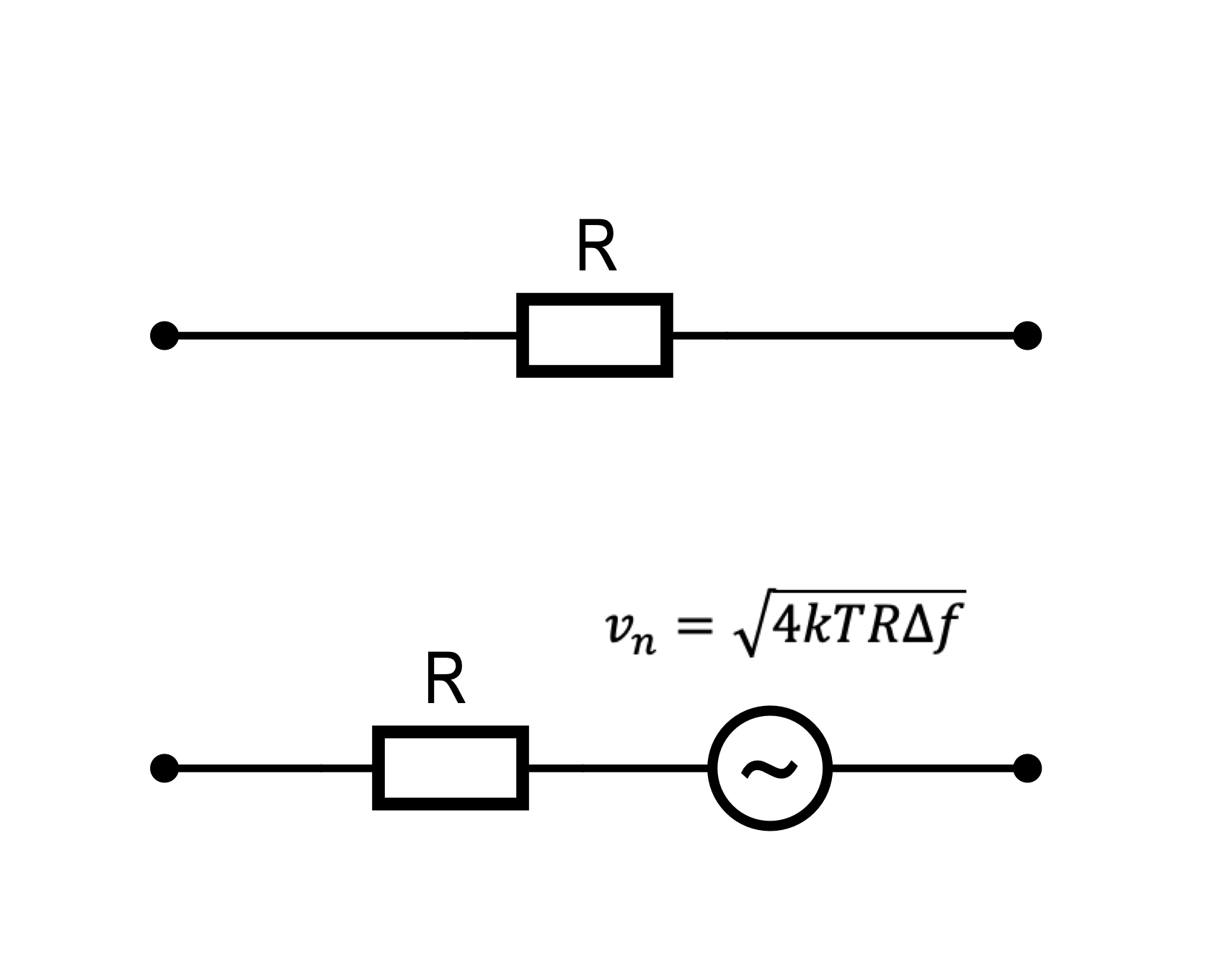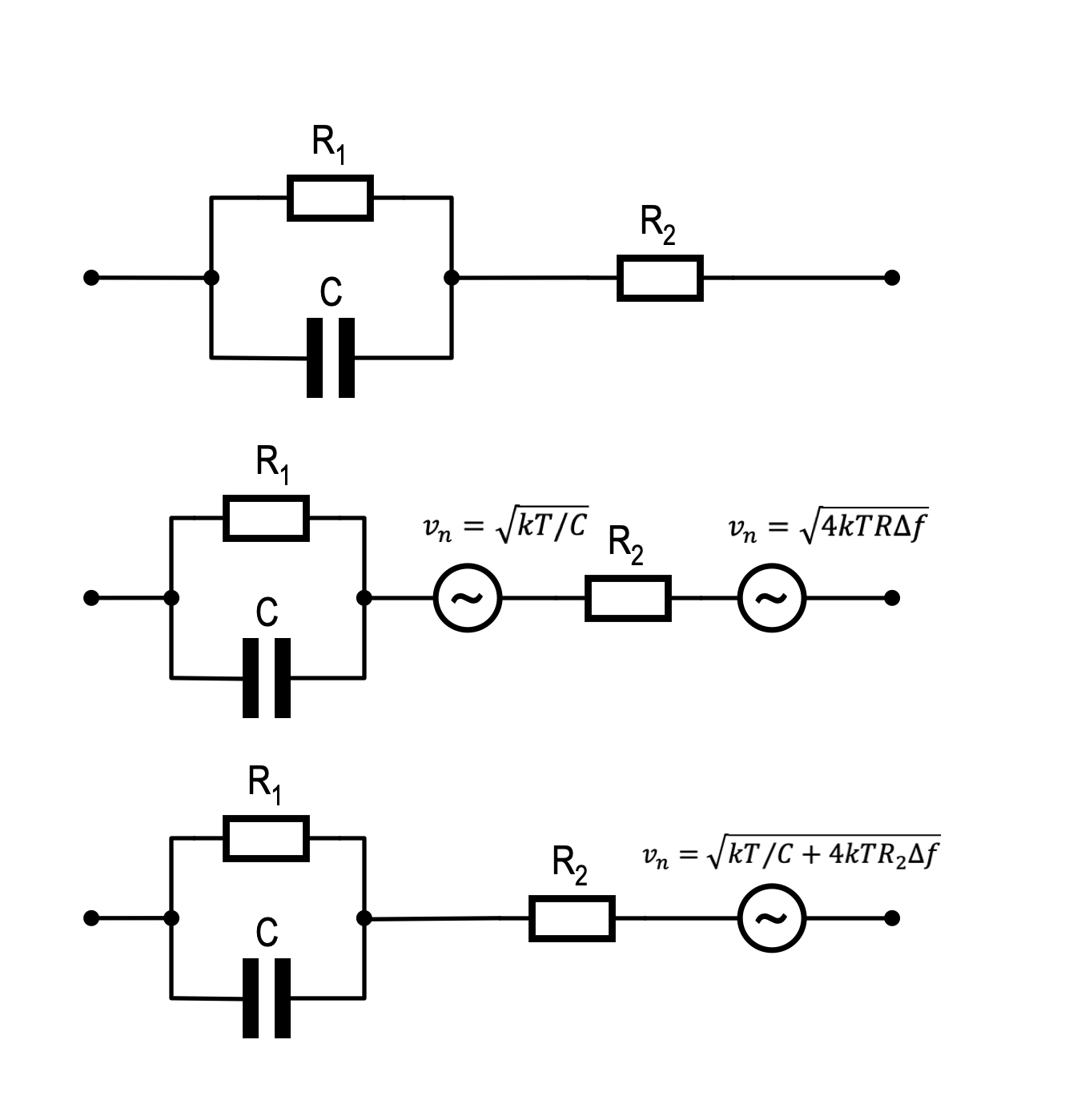# Noise in Passive CircuitsWritten by

All electronic circuits are affected by noise which is defined the unwanted disturbances in an electronic signal caused by physical phenomenon in the components themselves. This is distinct from unwanted disturbances that might be couples into a circuit from external sources. There are various types of noise in electronics, each caused by a different phenomenon and each with unique characteristics.

This article deals with noise in passive components where the predominant source Johnson noise, sometimes also called Nyquist or thermal noise. Johnson noise is essentially the electrical signal produced in lossy components by the random movement of charge carriers (usually electrons) due to temperature. For example, a 10kΩ resistor just sitting unconnected on your bench at room temperature (~300K) will develop a noise voltage across the terminals of around 13µVrms if measured with a voltmeter with a 1MHz bandwidth.

This highlights to the first important thing to keep in mind when discussing noise – we can only specify a noise voltage or current if we also specify a bandwidth over which to measure it. Since Johnson noise is a wideband phenomenon with equal energy across the frequency spectrum right up to the Terahertz range, we normally specify it in terms of a voltage or current per unit frequency, called the voltage of current noise density. Noise densities are expressed in units of voltage or current per square root Hz. You just multiply the voltage or current noise density by the square root of the bandwidth to get the rms voltage or current.

In the case of Johnson noise, the noise voltage density in a resistor is given by the equation below, where k is Boltzmann’s constant (1.38 × 10-23), T is the temperature in Kelvin and R is the resistance in Ohms.

Recognising that noise voltage density is in units of volts per root Hz, we can rearrange things to get an equation for the noise voltage by including the bandwidth f.

Figure 1 shows that the noise equivalent circuit of a resistor is a theoretical noiseless resistor in series with an AC source with a voltage described by the equation above. You can normally ignore the noise generated by conductors since their resistance is usually so low that any noise that they may contribute is negligible. In fact, lossless devices in general do not contribute to the overall noise in a circuit.Figure 1A simple resistor will develop a noise voltage across it (and a corresponding current through it) due to the movement of electrons due to temperature. The resistor can be considered to be equivalent to a noiseless resistor in series with an AC voltage source with rms value given by the equation shown. The noise voltage is dependant on the resistor value, the temperature and the bandwidth over which we measure it.

Lossless devices in this case includes capacitors and inductors. While these do not exhibit Johnson noise themselves, they do influence the bandwidth of the circuit and so can modify the noise voltages or currents generated elsewhere. Consider a parallel RC circuit a shown in Figure 2. The bandwidth is limited by the RC time constant as shown below. Substituting this into the equation for Johnson noise gives an expression for the noise voltage.

Δf=1/4RC ∴ v_n=√(kT/C)Figure 2In the case of a parallel RC circuit, the noise voltage is related to the temperature and capacitance value. This is somewhat counterintuitive since the resistor is the sole source of noise. The RC combination sets the bandwidth of the noise, cancelling out the resistance term in the equation for Johnson noise leaving the noise voltage related only to capacitance and temperature. Increasing the resistor value increases its noise density but reduces the bandwidth proportionally, leaving the noise voltage independent of resistance.

Notice that the value of the resistor has cancelled out even though it is the source of the noise, and the bandwidth term has disappeared since the circuit itself limits the bandwidth. This slightly counterintuitive result suggests that the noise voltage of a parallel RC circuit is related only to the temperature and the capacitor value. For example, placing a 100nF capacitor across the 10kΩ resistor in our first example, reduces the noise voltage from 13µV to just 203nV.

When considering noise in circuits, an important thing to keep in mind is how the noise in multiple sources accumulates. As long as the noise sources are not correlated, and the Johnson noise in any two components should not be, the noise voltage or voltage densities add as the root of the sum of squares.

Imagine add a second 1kΩ resistor in series with our parallel 10kΩ/100nF combination. The 1 MHz bandwidth noise voltage across this new resistor will be around 4.1µV at room temperature and that from the original RC combination is 203nV. The noise from the series combination is the root sum of squares which works out to be around 4.1µV since the noise in the 1kΩ resistor dominates. The idea of a dominant noise source is quite common and allows us to simplify noise calculations in many situations. Figure 3 shows the equivalent circuit and the resulting generalised noise voltageFigure 3Adding a resistor in series with the parallel RC combination means we need to sum the noise voltages generated. Since noise voltages are uncorrelated random fluctuations with a mean of zero, we sum them by calculating the root sum of squares.

In summary, for passive components we need only be concerned with Johnson noise which arises from thermal effects. It is described by a noise density function and requires a defined bandwidth in order to be expressed as a voltage or a current. Only lossy components like resistances are sources of Johnson noise, although capacitors and inductors can shape the noise due to their impact on bandwidth. Uncorrelated noise sources add according to the root sum of squares.

Armed with this information, we can tackle most passive noise calculations. Once we introduce semiconductors into the mix, we have to consider Johnson noise plus a few new types of noise with different characteristics. This is a whole new topic for another day.

References
“Johnson–Nyquist Noise.” In Wikipedia, August 26, 2022. https://en.wikipedia.org/w/index.php?title=Johnson%E2%80%93Nyquist_noise&oldid=1106753708.

Horowitz, Paul, and Winfield Hill. The Art of Electronics. Third edition, 11th printing, with Corrections. Cambridge New York, NY: Cambridge University Press, 2017.

 Keep up-to-date with our FREE Weekly Newsletter! Don't miss out on upcoming issues of Circuit Cellar. Subscribe to Circuit Cellar Magazine Note: We’ve made the May 2020 issue of Circuit Cellar available as a free sample issue. In it, you’ll find a rich variety of the kinds of articles and information that exemplify a typical issue of the current magazine. Would you like to write for Circuit Cellar? We are always accepting articles/posts from the technical community. Get in touch with us and let's discuss your ideas.